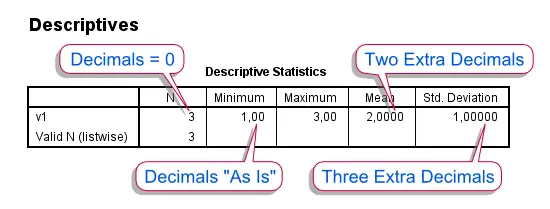SPSS TUTORIALS BASICS ANOVA REGRESSION FACTOR CORRELATION

# Set Decimals for Output

Setting decimal places in SPSS output is not straightforward. A great option, however, is our Set Decimals for Output Tables Tool. An alternative is changing your variable formats. Finally, for full control, one needs Python Scripting or SaxBasic.

## 1. Changing Variable Formats

The number of decimals in SPSS output basically depends on the combination of statistic, procedure and variable format. Manipulating the latter is usually the easiest way to control the output formats to some extent. For example, try and run the following syntax.
*1. Specify format for new numeric variables.

set format=f8.2.

*2. Create mini test data.

data list free/v1.
begin data
1 2 3
end data.

*3. Basic descriptives.

descriptives v1.

*4. Change variable format.

format v1(f2.0).

*5. Basic descriptives.

descriptives v1.
By comparing the first and second descriptives tables, four different rules for the number of decimals can be derived:
• N always has zero decimals (which sort of makes sense)
• Minimum and maximum take their numbers of decimals directly from the variable format
• The mean has two extra decimals
• The standard deviation has three extra decimalsSPSS Output - Decimal Places for Descriptives

## 2. Using Python Scripting

As illustrated, some control over decimals can be exerted by changing variable formats. However, this is rather limited. For instance, the mean wil always have at least two decimals and the standard deviation will always have one decimal more than the mean. These limitations can be overcome with either SaxBasic (which is deprecated) or Python Scripting. A very basic Python script for setting all decimal places to zero is
*Set all decimal places in a "descriptives" table to zero.

begin program.
import SpssClient
SpssClient.StartClient()
oDoc = SpssClient.GetDesignatedOutputDoc()
oItems = oDoc.GetOutputItems()
for index in range(oItems.Size()):
oItem = oItems.GetItemAt(index)
if oItem.GetDescription() == "Descriptive Statistics" and oItem.GetType() == SpssClient.OutputItemType.PIVOT:
pTable = oItem.GetSpecificType()
dCells=pTable.DataCellArray()
for rows in range(dCells.GetNumRows()):
for cols in range(dCells.GetNumColumns()):
dCells.SetHDecDigitsAt(rows,cols,0)
SpssClient.StopClient()
end program.
Although this basic script is a bit lengthy, modifying it (for instance, have different decimal places for different statistics) wouldn't require too much extra code. Also, note that the script could be rewritten as a function (accepting parameters such as decimal places) and placed in a module for easier access.

## 3. The MODIFY TABLES Extension

An alternative way to fine tune the numbers of decimal places in output tables is to use the MODIFY TABLES extension, which can be downloaded here. This extension can do much more than setting decimal places but some find it hard to use (perhaps even harder than basic Python scripting). Under the hood it basically generates and runs a Python script (like the one we just saw) given some specifications. Setting decimal places to one for the Mean and Standard deviation is done like so
*Set decimals of descriptives to 1 with the modify tables extension.

SPSSINC MODIFY TABLES subtype="'Descriptive Statistics'"
SELECT="Mean" "Std. Deviation"
DIMENSION= COLUMNS
LEVEL = -1 PROCESS = ALL
/STYLES APPLYTO=DATACELLS
CUSTOMFUNCTION="customstylefunctions.SetDecimalPlaces(decimals=1)".

# Tell us what you think!

*Required field. Your comment will show up after approval from a moderator.

# THIS TUTORIAL HAS 4 COMMENTS:

•### By Keks on March 22nd, 2014

For SPSS Statistics 22 and later, there is a command "OUTPUT MODIFY" that can set decimals and many other things for output. Here is an example to set all cells of tables' decimals to "F.2" format:

OUTPUT MODIFY
/REPORT PRINTREPORT=NO
/SELECT TABLES
/DELETEOBJECT DELETE=NO
/OBJECTPROPERTIES VISIBLE=ASIS
/TABLECELLS SELECT=[BODY] SELECTCONDITION=ALL FORMAT="F.2" APPLYTO=CELL.

•### By admin on March 22nd, 2014

Dear Keks,

Thank you for pointing that out. As we write, we're not on version 22 yet but this looks promising!

Ruben

•### By Linda Martell on July 15th, 2015

Good

•### By Caroline on January 26th, 2016

Thanks for the assistance it works well